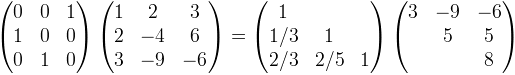﻿LUDecomposition Class# LUDecomposition Class

Represents the LU decomposition of a square matrix.Inheritance Hierarchy
SystemObject
Meta.Numerics.MatricesLUDecomposition

Namespace:  Meta.Numerics.Matrices
Assembly:  Meta.Numerics (in Meta.Numerics.dll) Version: 4.1.4Syntax
`public sealed class LUDecomposition`

The LUDecomposition type exposes the following members.Properties
NameDescriptionDimension
Gets the dimension of the system.
TopMethods
NameDescriptionDeterminant
Computes the determinant of the original matrix.Equals
Determines whether the specified object is equal to the current object.
(Inherited from Object.)GetHashCode
Serves as the default hash function.
(Inherited from Object.)GetType
Gets the Type of the current instance.
(Inherited from Object.)Inverse
Computes the the inverse of the original matrix.LMatrix
Gets the L factor.PMatrix
Gets the permutation matrix.Solve
Solves A x = b.ToString
Returns a string that represents the current object.
(Inherited from Object.)UMatrix
Gets the U factor.
TopRemarks

An LU decomposition is a representation of a matrix M as the product of a lower-left-triangular matrix L and and an upper-right-triangular matrix U. To reduce numerical instabilities, we actually decompose a row-wise permutation of a matrix, so that we have P A = L U, where P is a permutation matrix.

For example, here is an LU decomposition of a permutation of a simple 3 X 3 matrix:Given an LU decomposition of a permutation A, we can solve systems of linear equations or compute the determinant or inverse of A. LU decomposition is the fastest way to solve an arbitrary system of linear equations. It is much faster, and less subject to rounding errors, to solve Ax=b by LU decomposition than than by inverting A and multiplying A-1b.

You can use the LUDecomposition method to obtain the LU decomposition of any non-singular square matrix.See Also